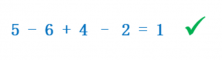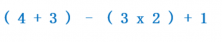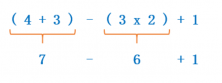Smartick is a fun way to learn math!Mar23

# Learn How to Perform Combined Operations

Do you want to learn how to perform combined operations? On the Smartick blog, we have already talked about combined operations on other occasions, but now we will look at more examples and we will solve them together. Shall we start?

### What are combined operations?

Combined operations are numerical expressions in which multiple operations (addition, subtraction, multiplication or division) are displayed with parentheses, brackets, or more.

### How do we perform combined operations?

To perform combined operations you have to follow a few simple steps:

1. Perform the operation or operations within the parentheses first.

2. If there are several operations in a row, complete the multiplication and division first and then addition and subtraction.

Solved examples of combined operations

Let’s see 3 examples, starting with the easiest.

## 5 – 3 x 2 + 4 – 4 ÷ 2

In this case, since there are no parentheses we have to look at the operations: first, find all of the multiplication and division that appears:

5 – 3 x 2 + 4 – 4 ÷ 2

Once we have identified them, we must perform the operations:

5 – 3 x 2 + 4 – 4 ÷ 2

5 – 6 + 4 – 2

Now there are only addition and subtraction left, so we evaluate the expression:### Example 2:In this example, there are parentheses, so we first have to perform the operations inside them:Now we look at the remaining operations, which are only addition and subtraction. Therefore, we can operate from left to right and evaluate the expression:

## 3 x (4 x 2 – 3) – (4 + 6 ÷ 3)

In this example, we have parentheses. Therefore, we have to perform the operations within them. Watch out! Within the parentheses there are several operations, so we first have to look at the multiplication and division within the parentheses:

3 x (4 x 2 – 3)(4 + 6 ÷ 3)

Once we know which operations we have to do first, we can calculate them:

3 x (4 x 2 – 3) – (4 + 6 ÷ 3)

3 x (8 – 3) – (4 + 2)
Now, within the parentheses, there is only one operation we can perform:

3 x (8 – 3) (4 + 2)

3 x 5 6

Once the parentheses are gone, we return to the operations. First, you have to do multiplication:
3 x 5 – 6

15 – 6
Once the multiplication is done we can evaluate the expression:
15 – 6 = 9

What do you think of combined operations? They’re easy, right?

And if you want to continue learning more primary mathematics, go to Smartick and try it for free!

Fun is our brain’s favorite way of learning
Diane Ackerman
Smartick is a fun way to learn math
• 15 fun minutes a day